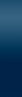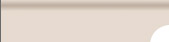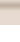HomeFitness articlesASP.Net articlesSEO (Search Engine Optimization) ArticlesGaming Articles## Using Arrays in ASP.NET 2.0

The use and need to temporarily store data in an organized way has always been around. Arrays have been one way to store this data in a nice and neat way. If you have experience with classic ASP, C++, or an older version of Visual Basic, using an array in ASP.NET should come pretty easily to you. This tutorial is written specifically for VB.Net, but converting this code to C# would be an easy task.

To use an array, you first need to declare the array. The array can be an array of any type. You can also set a specific size to the array if you wish. Here are a few examples of declaring the array:

Dim dblArray(100) As Double 'Declare an array of doubles of length 100
Dim dblArray1() As Double 'Declare an array of doubles with no length set
Dim strArray(100) As String 'Declare an strings of doubles of length 100
Dim strArray1() As string 'Declare an strings of doubles with no length set

Accessing and filling the array is very simple. To access a specific value in the array, we simply call the array with a specific index. One thing to remember is that the array is zero based. So, dblArray(0) is the first item in the array, and dblArray(19) is the 20th item in the array. Here is an example of how to fill and access an item in the array:

Dim iTempValue As Double 'Declare a Temporary variable.
dblArray(0) = 15 'Set the first item of the array equal to 15.
iTempValue = dblArray(0) 'Set iTempValue equal to the first item in the array which is 15.

The next thing that can be useful is filling an array dynamically. We don't always know how large the array needs to be, so we can use a counter variable along with the redim preserve command to fill an array dynamically. When doing this, we need to initially declare the array with no length. Here is an example

dim ArraySize as Long 'Declare our array counter
ArraySize = 0
While objDataReader.Read() 'Looping threw a Recordset, you can read Looping threw a Recordset to see how to do this.
ReDim Preserve dblArray1(ArraySize + 1) 'Redefine the array with a size of 1 larger than the last size
dblArray1(ArraySize) = objDataReader("double_value") 'Fill the array with the value from the DB
ArraySize = ArraySize + 1 'Increment the array size
End While

There are also many cool and helpful functions built into .Net for arrays.

'Sort the Array ascending
Array.Sort(dblArray1)

'Reverse the Array
Array.Reverse(dblArray1)

'Sort the Array descending
Array.Sort(dblArray1)
Array.Reverse(dblArray1)

'Copy the array or part of the array to another array
Array.Copy(dblArray1, dblArray, 5) 'Copies the first 5 elements of dblArray1 to dblArray

'Get the upper bound of an array. This is helpful if you need to loop threw an array
UBound(dblArray1)

'Here is an example of looping threw an array using the Ubound function.
For i = 0 to UBound(dblArray1)
dblArray1(i) = i
Next

Related Articles:

ASP.Net Home